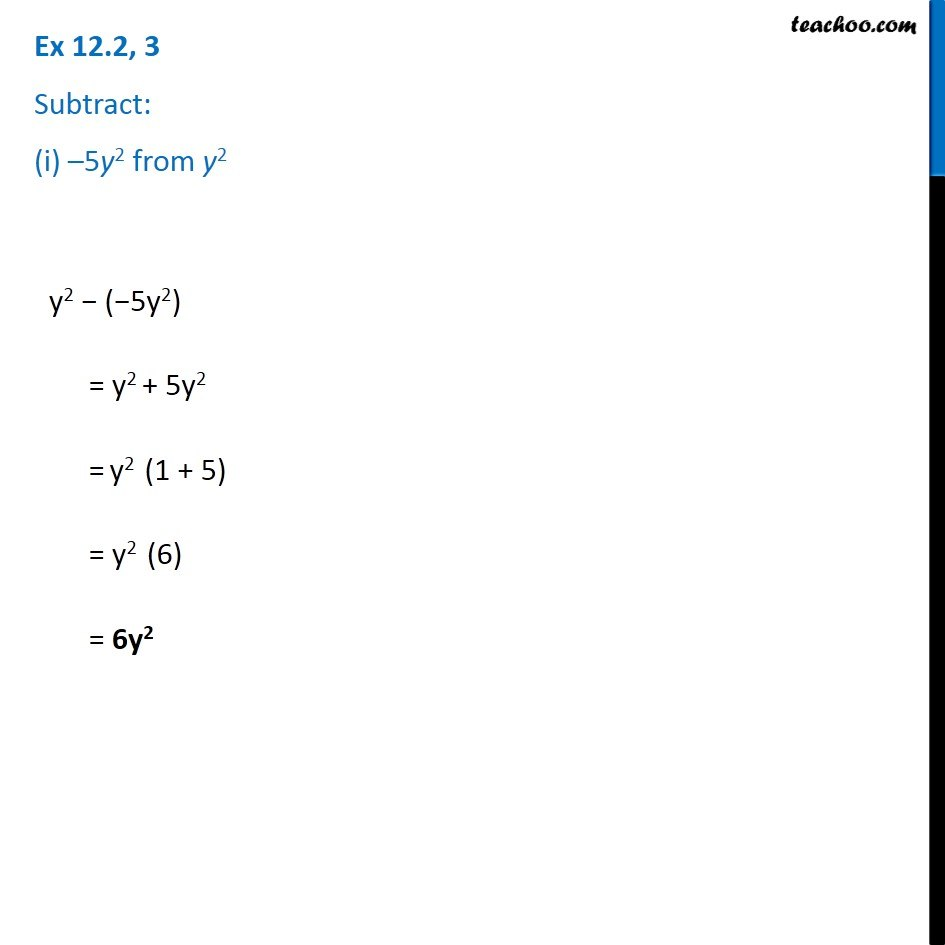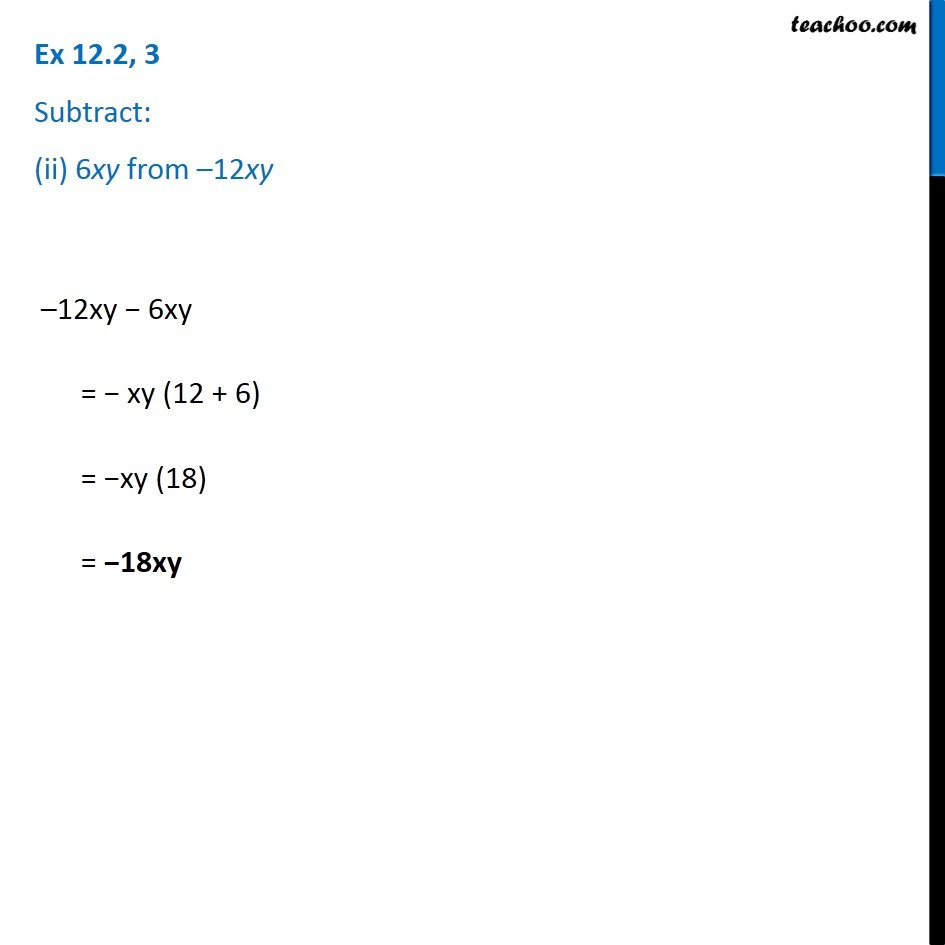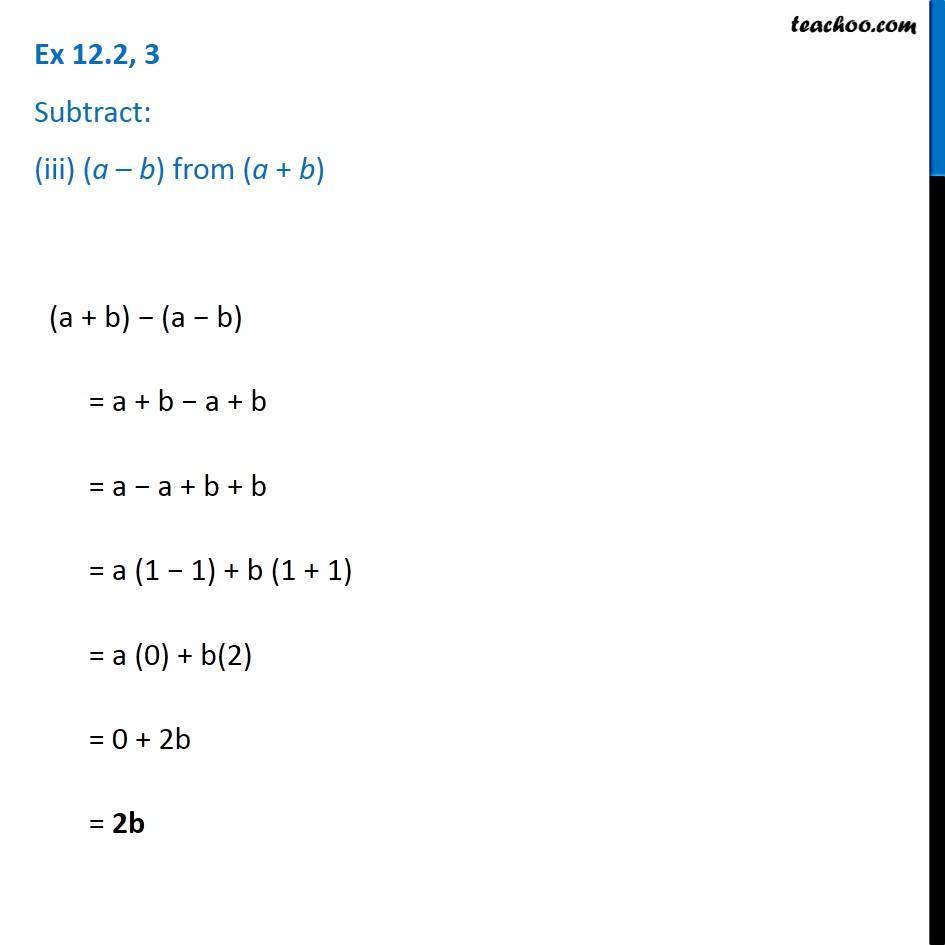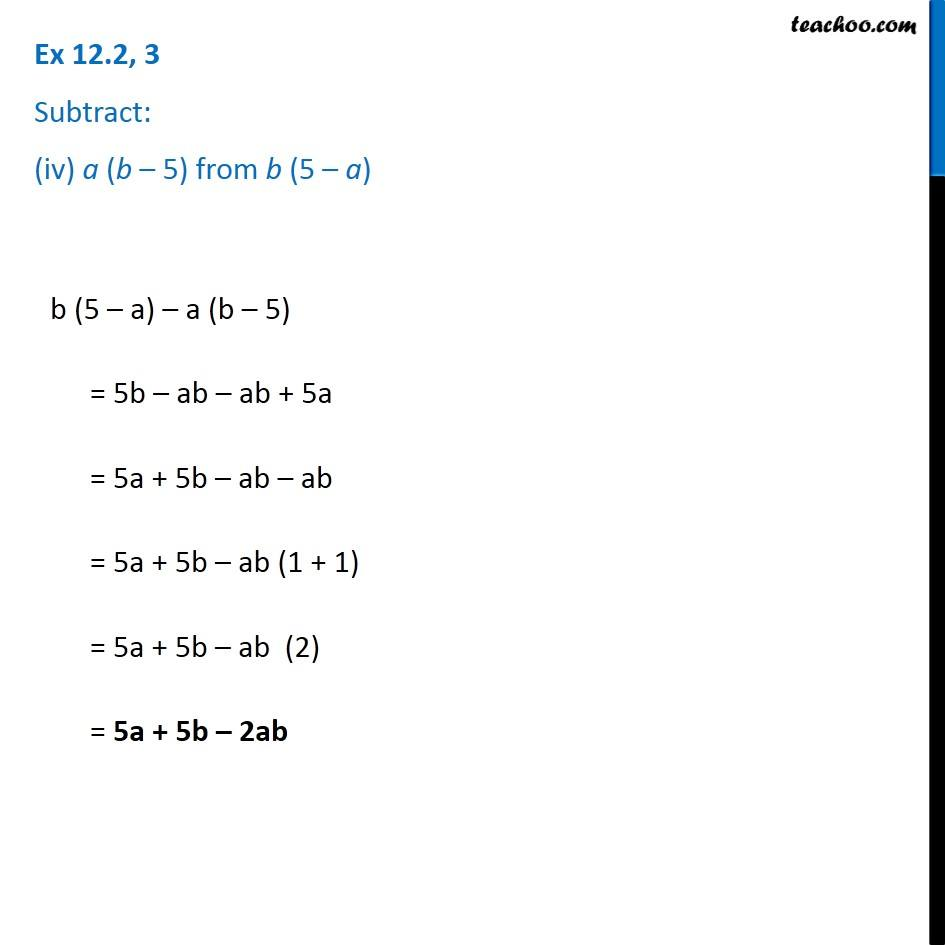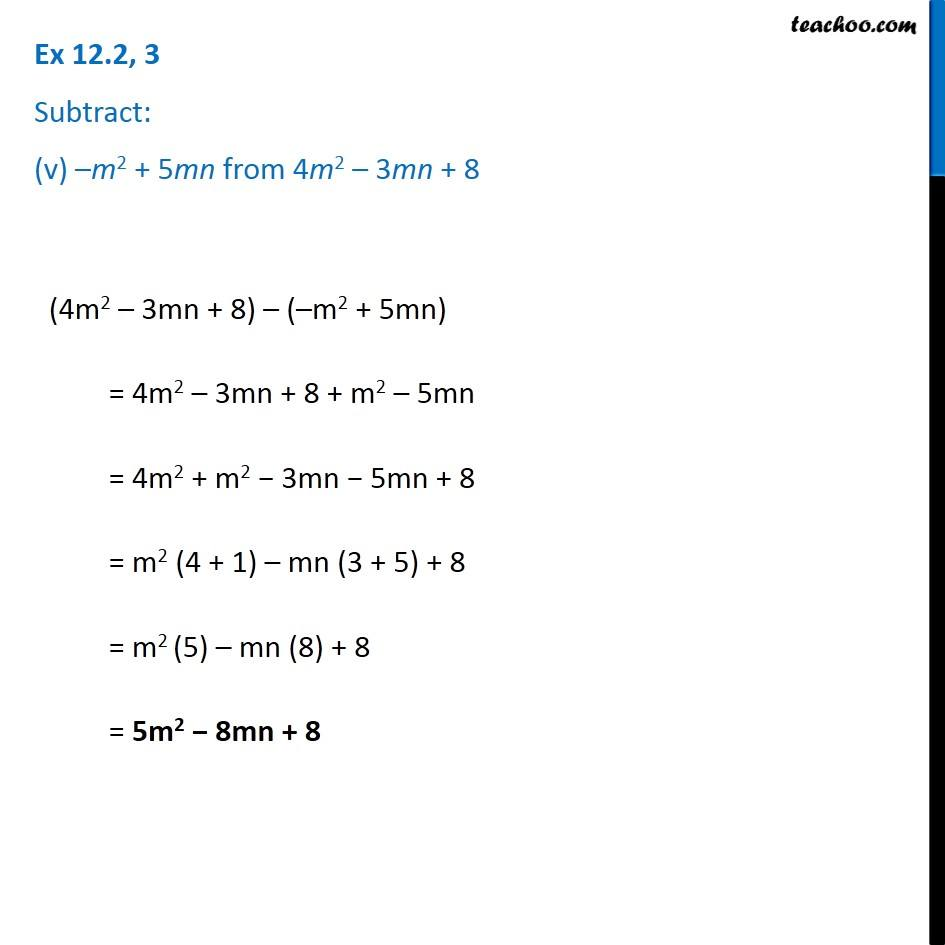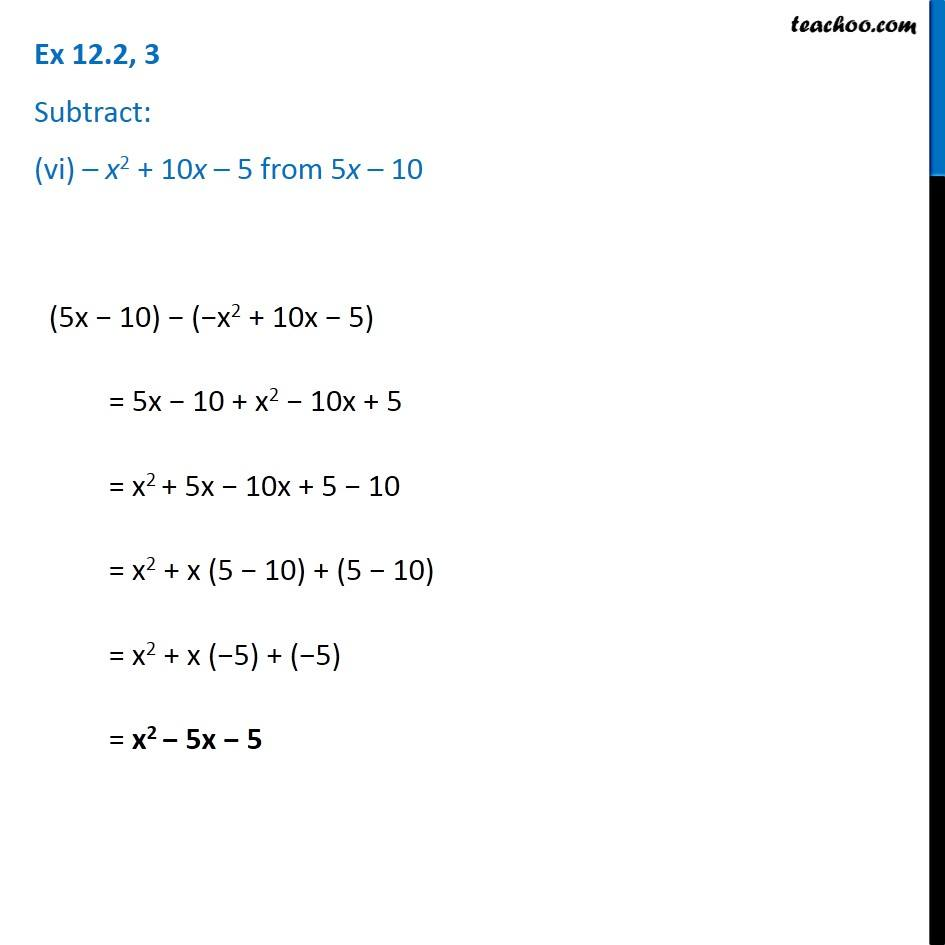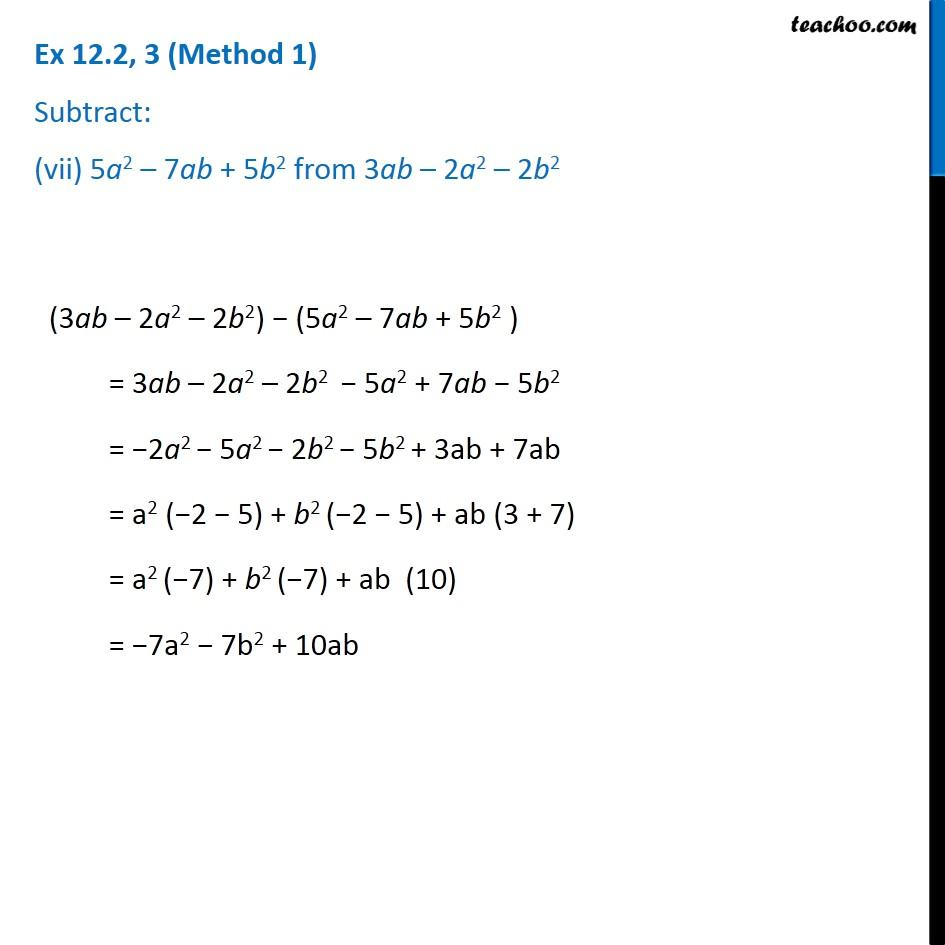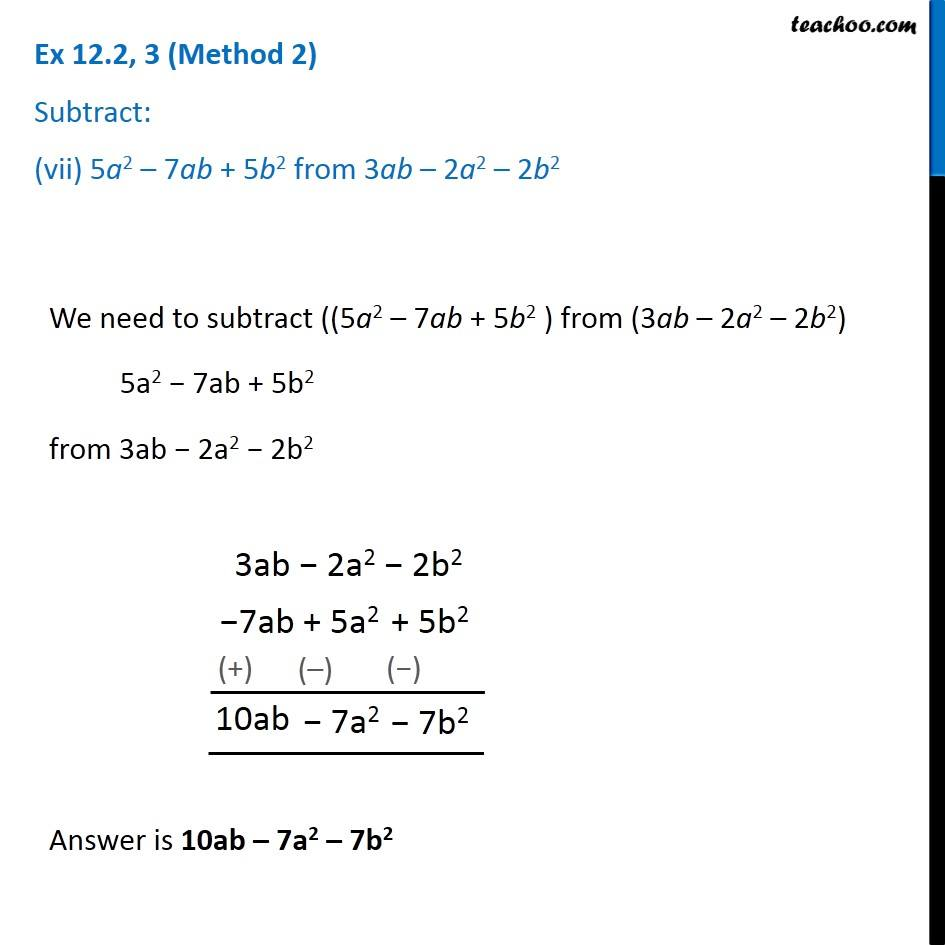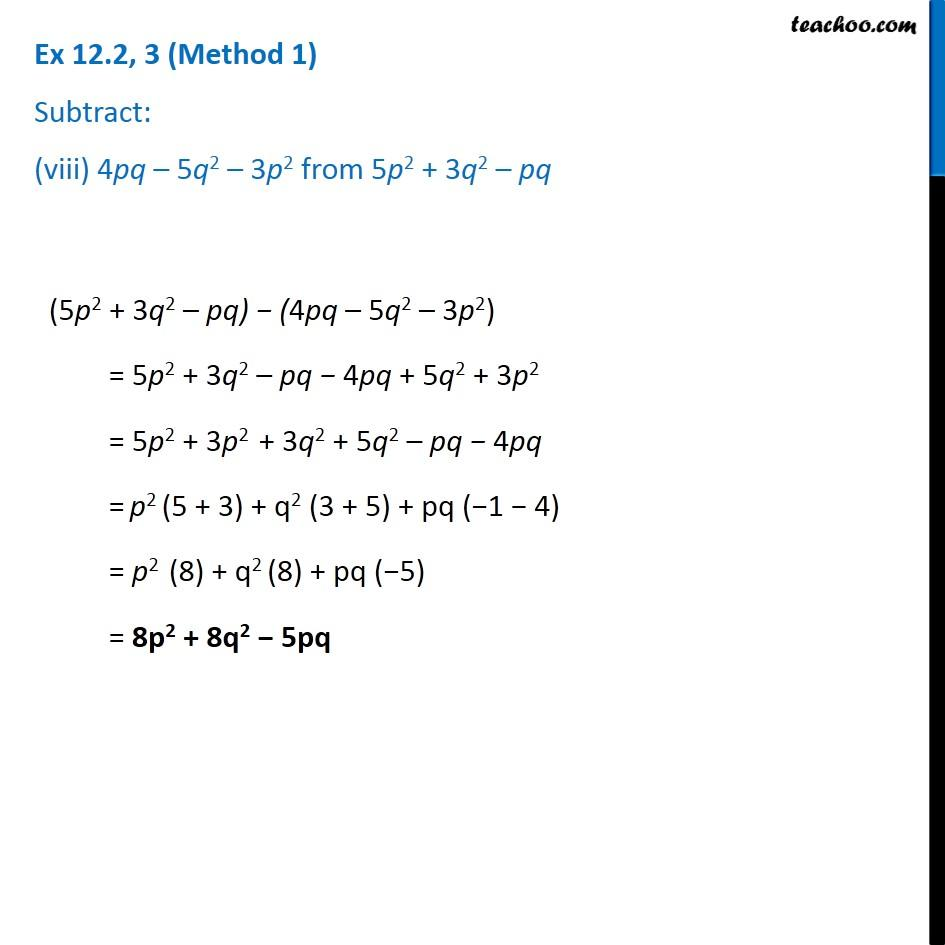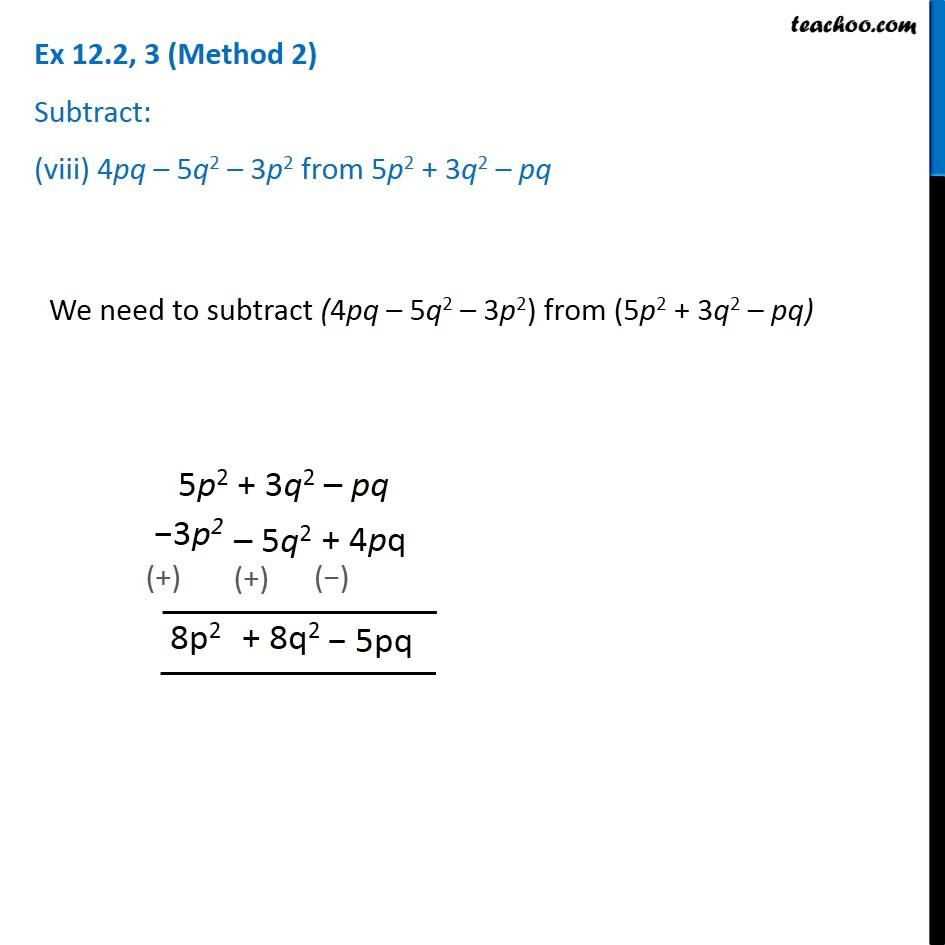Subscribe to our Youtube Channel - https://you.tube/teachoo

1. Chapter 12 Class 7 Algebraic Expressions
2. Serial order wise
3. Ex 12.2

Transcript

Ex 12.2, 3 Subtract: (i) –5y2 from y2 y2 − (−5y2) = y2 + 5y2 = y2 (1 + 5) = y2 (6) = 6y2 Ex 12.2, 3 Subtract: (ii) 6xy from –12xy –12xy − 6xy = − xy (12 + 6) = −xy (18) = −18xy Ex 12.2, 3 Subtract: (iii) (a – b) from (a + b) (a + b) − (a − b) = a + b − a + b = a − a + b + b = a (1 − 1) + b (1 + 1) = a (0) + b(2) = 0 + 2b = 2b Ex 12.2, 3 Subtract: (iv) a (b – 5) from b (5 – a) b (5 – a) – a (b – 5) = 5b – ab – ab + 5a = 5a + 5b – ab – ab = 5a + 5b – ab (1 + 1) = 5a + 5b – ab (2) = 5a + 5b – 2ab Ex 12.2, 3 Subtract: (v) –m2 + 5mn from 4m2 – 3mn + 8 (4m2 – 3mn + 8) – (–m2 + 5mn) = 4m2 – 3mn + 8 + m2 – 5mn = 4m2 + m2 − 3mn − 5mn + 8 = m2 (4 + 1) – mn (3 + 5) + 8 = m2 (5) – mn (8) + 8 = 5m2 − 8mn + 8 Ex 12.2, 3 Subtract: (vi) – x2 + 10x – 5 from 5x – 10 (5x − 10) − (−x2 + 10x − 5) = 5x − 10 + x2 − 10x + 5 = x2 + 5x − 10x + 5 − 10 = x2 + x (5 − 10) + (5 − 10) = x2 + x (−5) + (−5) = x2 − 5x − 5 Ex 12.2, 3 (Method 1) Subtract: (vii) 5a2 – 7ab + 5b2 from 3ab – 2a2 – 2b2 (3ab – 2a2 – 2b2) − (5a2 – 7ab + 5b2 ) = 3ab – 2a2 – 2b2 − 5a2 + 7ab − 5b2 = −2a2 − 5a2 − 2b2 − 5b2 + 3ab + 7ab = a2 (−2 − 5) + b2 (−2 − 5) + ab (3 + 7) = a2 (−7) + b2 (−7) + ab (10) = −7a2 − 7b2 + 10ab Ex 12.2, 3 (Method 2) Subtract: (vii) 5a2 – 7ab + 5b2 from 3ab – 2a2 – 2b2 We need to subtract ((5a2 – 7ab + 5b2 ) from (3ab – 2a2 – 2b2) 5a2 − 7ab + 5b2 from 3ab − 2a2 − 2b2 Answer is 10ab – 7a2 – 7b2 Ex 12.2, 3 (Method 1) Subtract: (viii) 4pq – 5q2 – 3p2 from 5p2 + 3q2 – pq (5p2 + 3q2 – pq) − (4pq – 5q2 – 3p2) = 5p2 + 3q2 – pq − 4pq + 5q2 + 3p2 = 5p2 + 3p2 + 3q2 + 5q2 – pq − 4pq = p2 (5 + 3) + q2 (3 + 5) + pq (−1 − 4) = p2 (8) + q2 (8) + pq (−5) = 8p2 + 8q2 − 5pq Ex 12.2, 3 (Method 2) Subtract: (viii) 4pq – 5q2 – 3p2 from 5p2 + 3q2 – pq We need to subtract (4pq – 5q2 – 3p2) from (5p2 + 3q2 – pq)

Ex 12.2